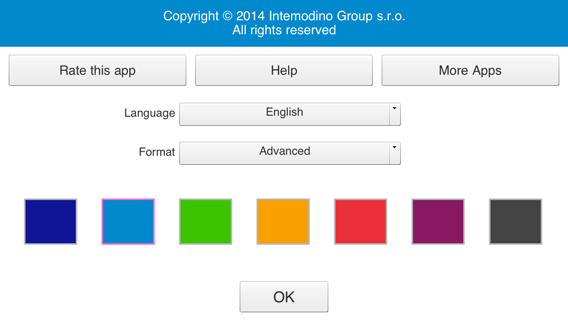# What equation format do I select for my equation?

The "Advanced" format with manual input can be used equally well for simple equations in the form ax + b = c and ax + b = cx + d and for more complex linear equations, including equations with parentheses, fractions, and decimals.

For linear equations in the forms ax + b = c and ax + b = cx + d you can also use the built-in templates.

You can select the necessary format in the Settings window.Monday, December 4, 2023

Contact